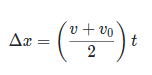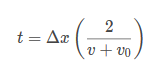hupfer

New member
Hello,
Could someone help me with a simple problem?
I would like to know how to solve a fraction within a fraction.
Example:
10/4/x

The issue I'm having is with a formula from a physics problem. I have this formulaand I need to rearrange like this. I don't quite understand how to get there.

pka

Elite Member
The issue I'm having is with a formula from a physics problem. I have this formula View attachment 12181
I need to rearrange like this View attachment 12182. I don't quite understand how to get there.
\displaystyle \begin{align*}\Delta x &= \left( {\frac{{v + {v_0}}}{2}} \right)t\\{\left( {\frac{{v + {v_0}}}{2}} \right)^{ - 1}}\Delta x &= t\\\left( {\frac{2}{{v + {v_0}}}} \right)\Delta x &= t \end{align*}

•topsquark

hupfer

New member
Would you care to elaborate a little bit more?
I'm having trouble understanding how you got the negative exponent

Riman643

New member
Hello,
Could someone help me with a simple problem?
I would like to know how to solve a fraction within a fraction.
Example:
10/4/x
In this example the answer would be $$\displaystyle \frac{10x}{4}$$ which can be simplified to $$\displaystyle \frac{5x}{2}$$. When dividing a fraction by a number, you keep the denominator the same (in this case 4) and multiply the numerator (10) by the number on the bottom (x).

The issue I'm having is with a formula from a physics problem. I have this formula View attachment 12181 and I need to rearrange like this View attachment 12182. I don't quite understand how to get there.
For this physics equation, you want to isolate t, so you need to divide both sides by $$\displaystyle \frac{v + v_{o}}{2}$$. This will give you $$\displaystyle t = \frac{Δx}{\frac{v + v_{o}}{2}}$$. Using the same technique as above, we keep the denominator the same (v + vo) and multiply the numerator (Δx) by the number on the bottom (2). This will give you $$\displaystyle t = \frac{2 Δx}{v + v_{o}}$$ or as your equation shows $$\displaystyle t = Δx(\frac{2}{v + v_{o}})$$. I hope this clears up some of the confusion.

•topsquark

Subhotosh Khan

Super Moderator
Staff member
Hello,
Could someone help me with a simple problem?
I would like to know how to solve a fraction within a fraction.
Example:
10/4/x

The issue I'm having is with a formula from a physics problem. I have this formula View attachment 12181 and I need to rearrange like this View attachment 12182. I don't quite understand how to get there.
$$\displaystyle Δx=\left(\dfrac{v+v_0}{2}\right)t$$

Multiply both sides by 2 - to get,,

$$\displaystyle 2 * Δx = (v+v_0) * t$$

can you continue?

•topsquark